Next: Forced precession and nutation Up: Rigid body rotation Previous: Free precession of Earth

# MacCullagh's formula

According to Equations (3.59) and (3.64), if the Earth is modeled as spheroid of uniform density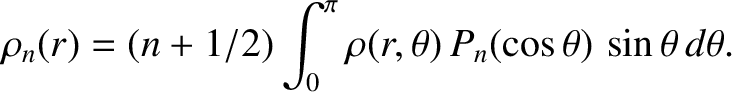then its ellipticity is given by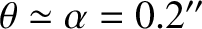(8.65)

where the integral is over the whole volume of the Earth, and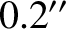would be the Earth's moment of inertia were it exactly spherical. The Earth's moment of inertia about its axis of rotation is given by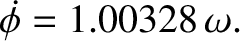(8.66)

Here, use has been made of Equations (3.24)-(3.26). Likewise, the Earth's moment of inertia about an axis perpendicular to its axis of rotation (and passing through the Earth's center) is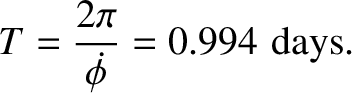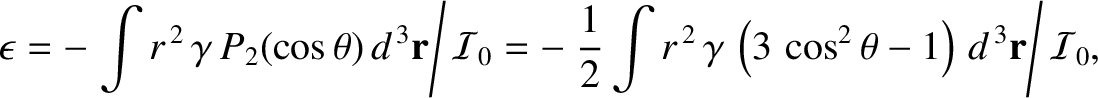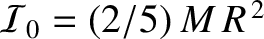(8.67)

because the average of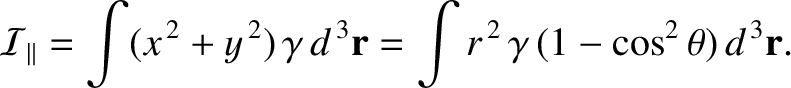is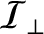for an axisymmetric mass distribution. It follows from the preceding three equations that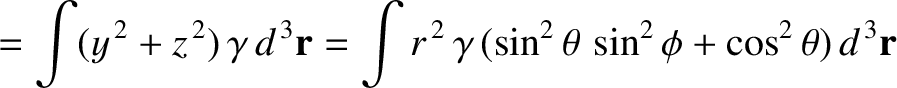(8.68)

When Equation (8.68) is combined with Equation (3.65), we get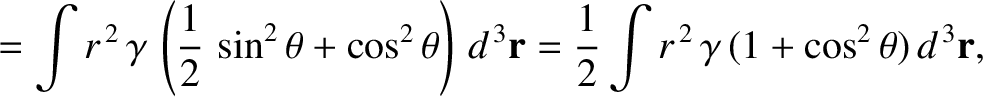(8.69)

which is the general expression for the gravitational potential generated outside an axially symmetric mass distribution. The first term on the right-hand side is the monopole gravitational potential that would be generated if all of the mass in the distribution were concentrated at its center of mass, whereas the second term is the quadrupole potential generated by any deviation from spherical symmetry in the distribution. Equation (8.69) actually holds for any axially symmetric mass distribution, not just a spheroidal mass distribution of uniform density (this is discussed in the following).

More generally, consider an asymmetric mass distribution consisting of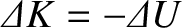mass elements. Suppose that the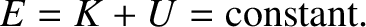th element has mass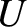and position vector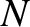, whereruns from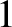to. Let us define a Cartesian coordinate system,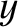,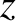such that the origin coincides with the center of mass of the distribution. It follows that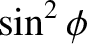(8.70)

Suppose that the-,-, and-axes coincide with the mass distribution's principal axes of rotation (for rotation about an axis that passes through the origin). It follows from Section 8.5 that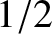(8.71)

and that the distribution's principal moments of inertia about the-,-, and-axes take the form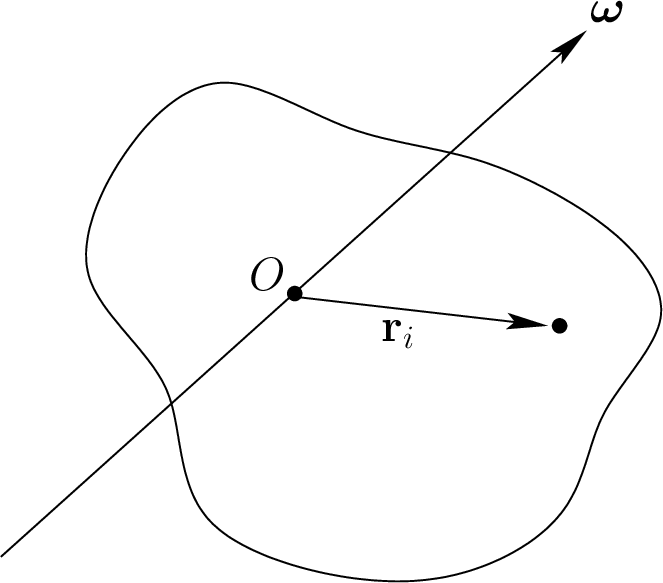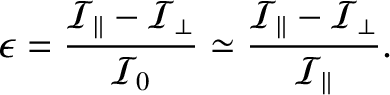(8.72)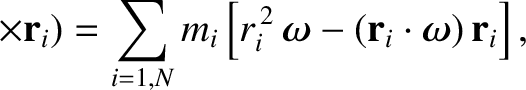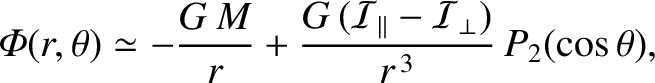(8.73) and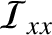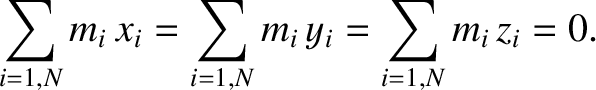(8.74)

respectively.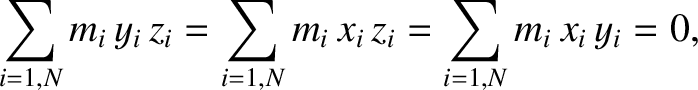Consider the gravitational potential,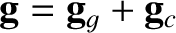, generated by the mass distribution at some external point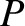whose position vector is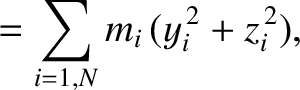,,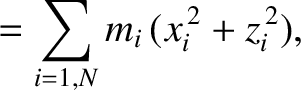. According to Section 3.2,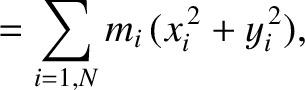(8.75)

which can also be written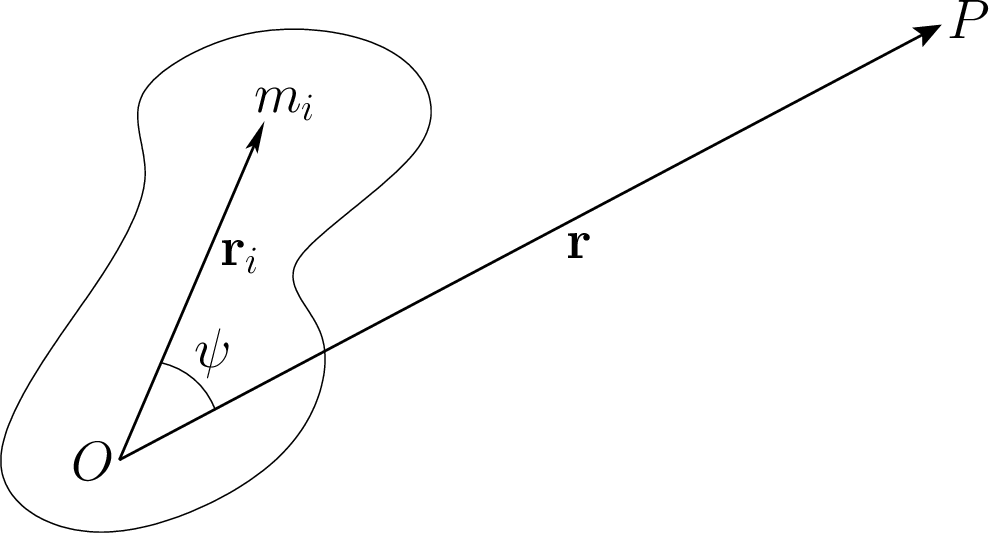(8.76)

where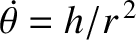is the angle subtended between the vectors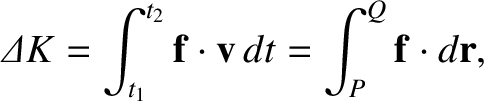and. (See Figure 8.3.) Suppose that the distance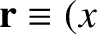is much larger than the characteristic radius of the mass distribution, which implies that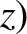for all. Expanding up to second order in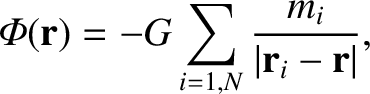, we obtain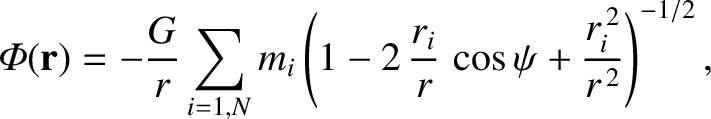(8.77)

However,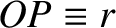(8.78)

where use has been made of Equations (8.70). Hence, we are left with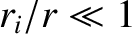(8.79)

Here,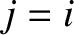is the total mass of the distribution. Now,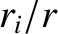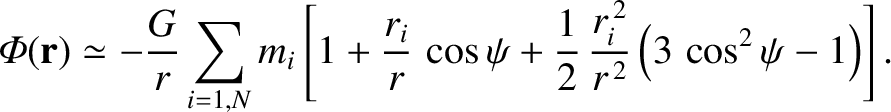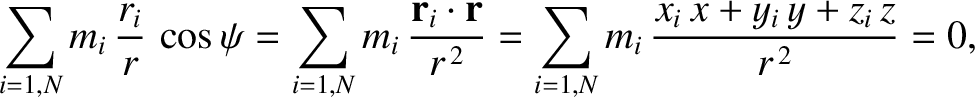(8.80)

where use has been made of Equations (8.72)-(8.74). Furthermore,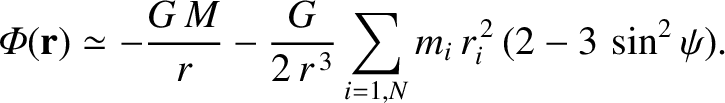(8.81)

is the distribution's moment of inertia about the axis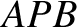. Thus, we deduce that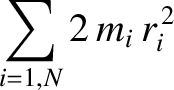(8.82)

This famous result is known as MacCullagh's formula, after its discoverer, the Irish mathematician James MacCullagh (1809-1847). Actually,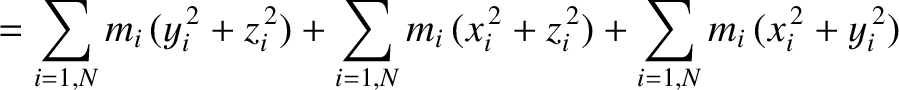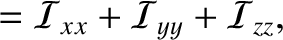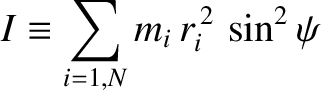(8.83)

Hence, MacCullagh's formula can also be written in the alternative form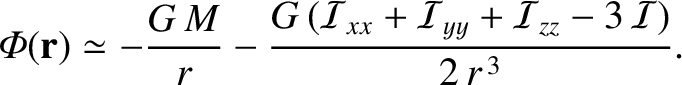(8.84)

Finally, for an axisymmetric distribution, such that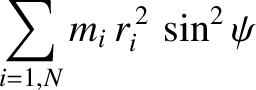and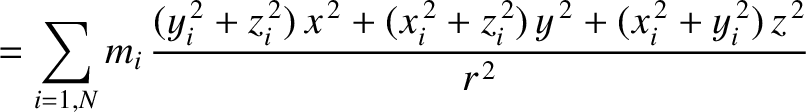, MacCullagh's formula reduces to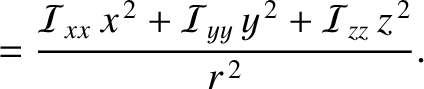(8.85)

where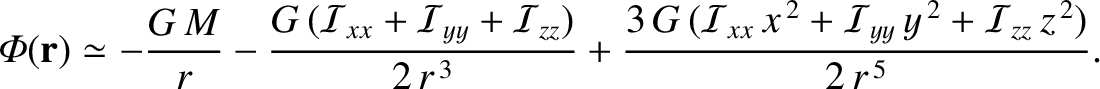. Of course, this expression is the same as Equation (8.69), which justifies our earlier assertion that this equation is is valid for a general axisymmetric mass distribution. Incidentally, a comparison of the preceding expression with Equation (3.66) reveals that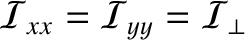(8.86)

where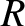is the mean radius of the distribution, and the dimensionless parameter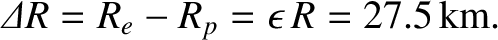characterizes the quadrupole gravitational field external to the distribution.Next: Forced precession and nutation Up: Rigid body rotation Previous: Free precession of Earth
Richard Fitzpatrick 2016-03-31